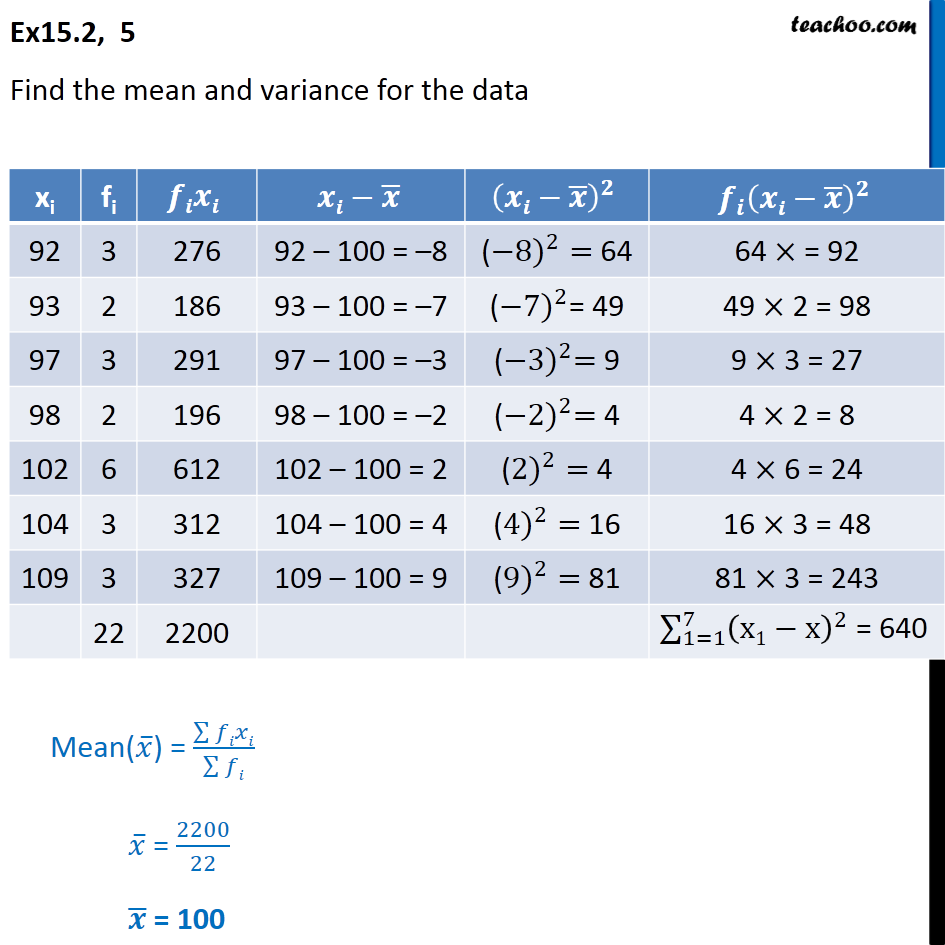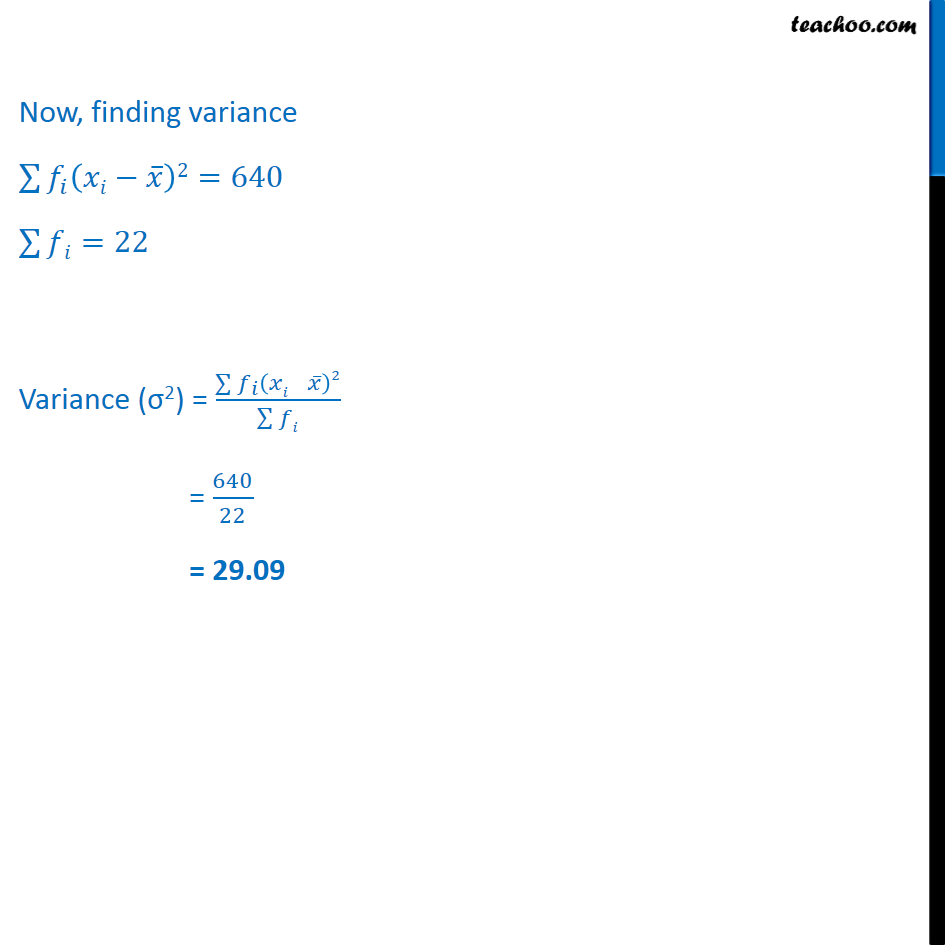Ex 13.2

Chapter 13 Class 11 Statistics
Serial order wiseLearn in your speed, with individual attention - Teachoo Maths 1-on-1 Class

### Transcript

Ex 13.2, 5 - Chapter 15 Class 11 Statistics - NCERT Find the mean and variance for the data xi fi fixi xi-x ̅ (xi-x ̅ )^2 〖f_i (xi-x ̅ )〗^2 92 3 276 92 – 100 = –8 (-〖8)〗^2= 64 64 × = 92 93 2 186 93 – 100 = –7 (〖-7)〗^2= 49 49 × 2 = 98 97 3 291 97 – 100 = –3 (〖-3)〗^2= 9 9 × 3 = 27 98 2 196 98 – 100 = –2 (〖-2)〗^2= 4 4 × 2 = 8 102 6 612 102 – 100 = 2 (〖2)〗^2= 4 4 × 6 = 24 104 3 312 104 – 100 = 4 (〖4)〗^2= 16 16 × 3 = 48 109 3 327 109 – 100 = 9 (〖9)〗^2= 81 81 × 3 = 243 22 2200 Mean(𝑥 ̅) = (∑▒𝑓𝑖𝑥𝑖)/(∑▒𝑓𝑖) 𝑥 ̅ = 2200/22 𝒙 ̅ = 100 Now, finding variance ∑▒〖𝑓_𝑖 (𝑥𝑖−𝑥 ̅ )2〗=640 ∑▒𝑓𝑖=22 Variance (σ2) = (∑▒〖𝑓_𝑖 (𝑥𝑖 − 𝑥 ̅ )2〗)/(∑▒𝑓𝑖) = 640/22 = 29.09